# Chemical Reactions Comprehensive Science Mr Knighton Chemical Reactions

• Slides: 26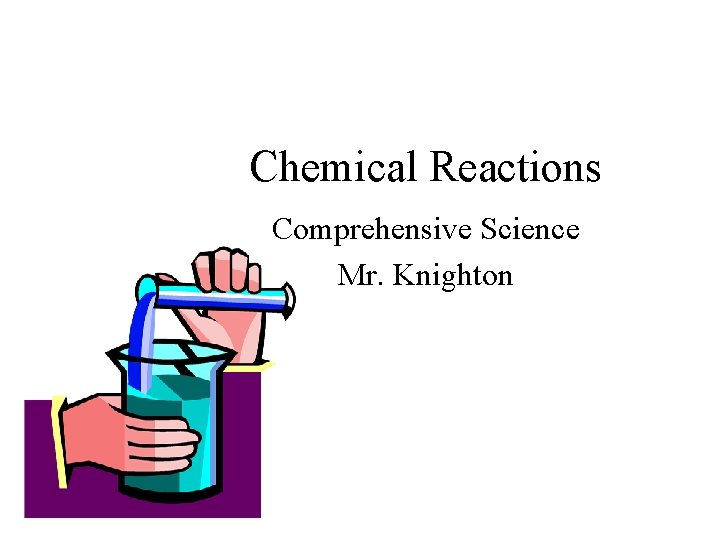Chemical Reactions Comprehensive Science Mr. KnightonChemical Reactions are all around us! Examples: • Growth, cooking, decaying, burning, …… Food + O 2 energy + CO 2 + H 2 O • This is a Chemical Reaction!! It is what takes place in your body when you eat!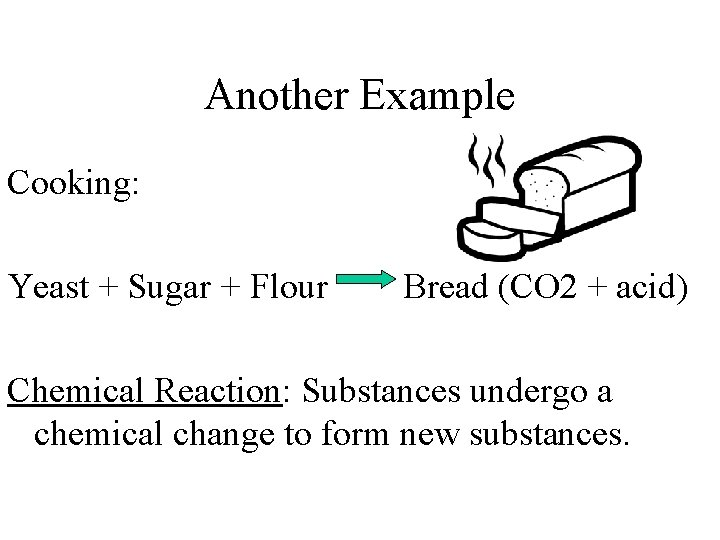Another Example Cooking: Yeast + Sugar + Flour Bread (CO 2 + acid) Chemical Reaction: Substances undergo a chemical change to form new substances.Signs of a Chemical Reaction • Production of gas – Ex) CO 2 bubbles are produced to make the bread rise. • Color Change – Ex) The White dough changes color to a golden brown after cooking.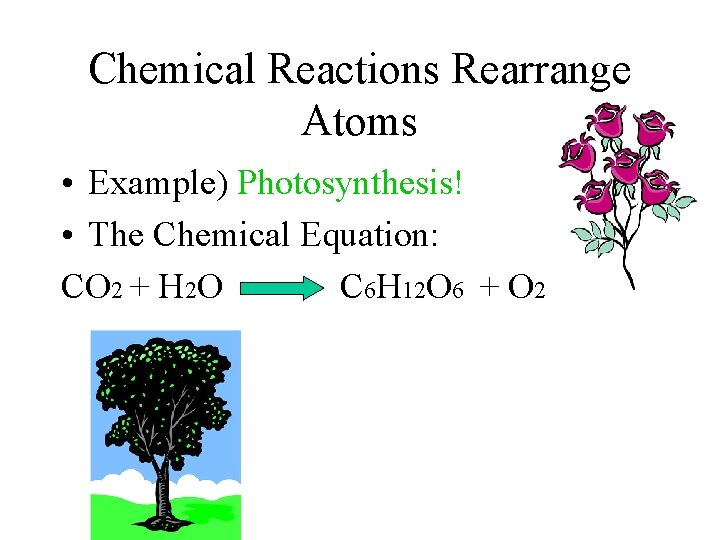Chemical Reactions Rearrange Atoms • Example) Photosynthesis! • The Chemical Equation: CO 2 + H 2 O C 6 H 12 O 6 + O 2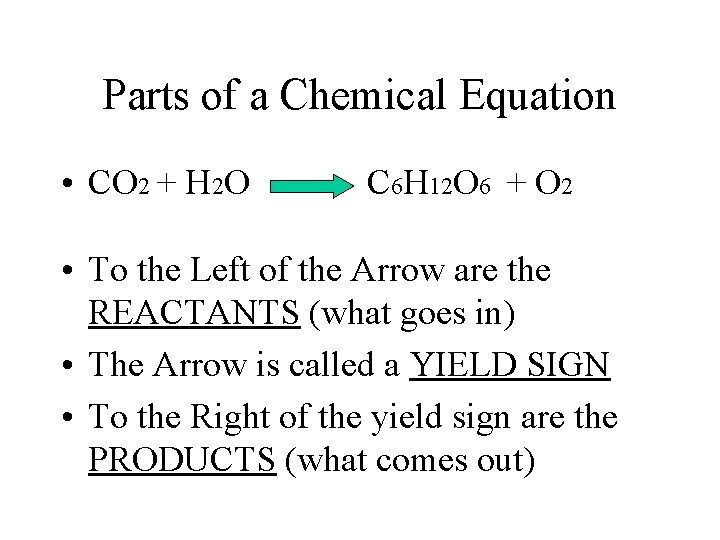Parts of a Chemical Equation • CO 2 + H 2 O C 6 H 12 O 6 + O 2 • To the Left of the Arrow are the REACTANTS (what goes in) • The Arrow is called a YIELD SIGN • To the Right of the yield sign are the PRODUCTS (what comes out)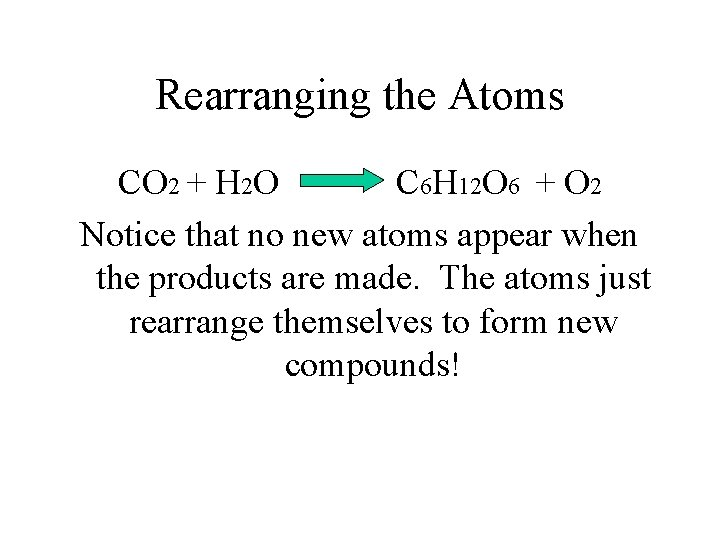Rearranging the Atoms CO 2 + H 2 O C 6 H 12 O 6 + O 2 Notice that no new atoms appear when the products are made. The atoms just rearrange themselves to form new compounds!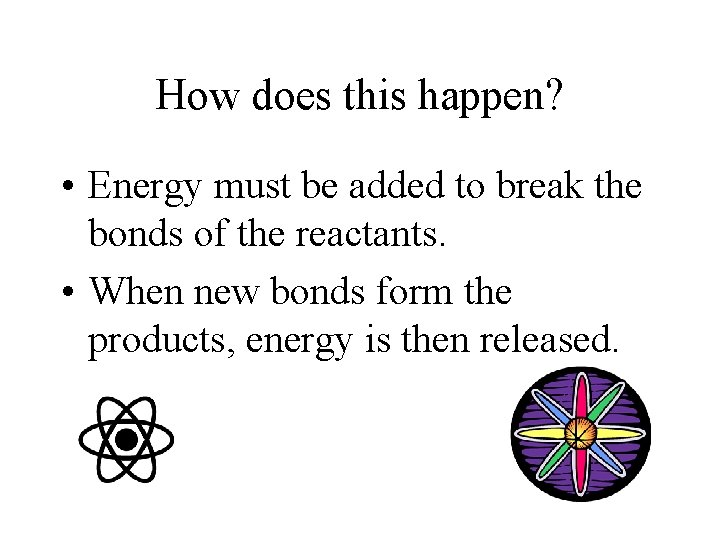How does this happen? • Energy must be added to break the bonds of the reactants. • When new bonds form the products, energy is then released.2 Major Types of Reactions • Exothermic: more energy is released then was absorbed. This produces HEAT! • Endothermic: More energy is abosrbed the released. Results in COLD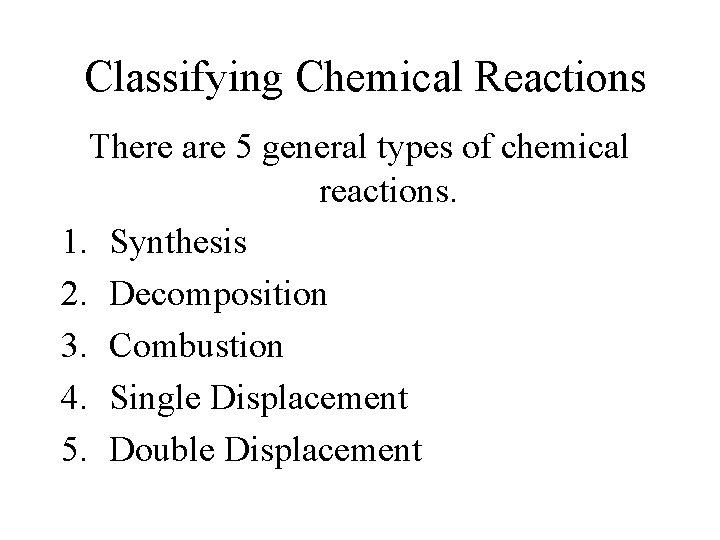Classifying Chemical Reactions There are 5 general types of chemical reactions. 1. Synthesis 2. Decomposition 3. Combustion 4. Single Displacement 5. Double Displacement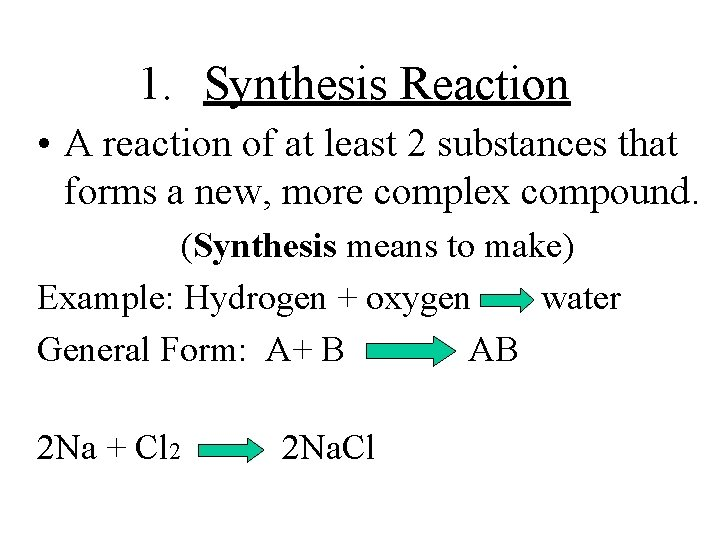1. Synthesis Reaction • A reaction of at least 2 substances that forms a new, more complex compound. (Synthesis means to make) Example: Hydrogen + oxygen water General Form: A+ B AB 2 Na + Cl 2 2 Na. Cl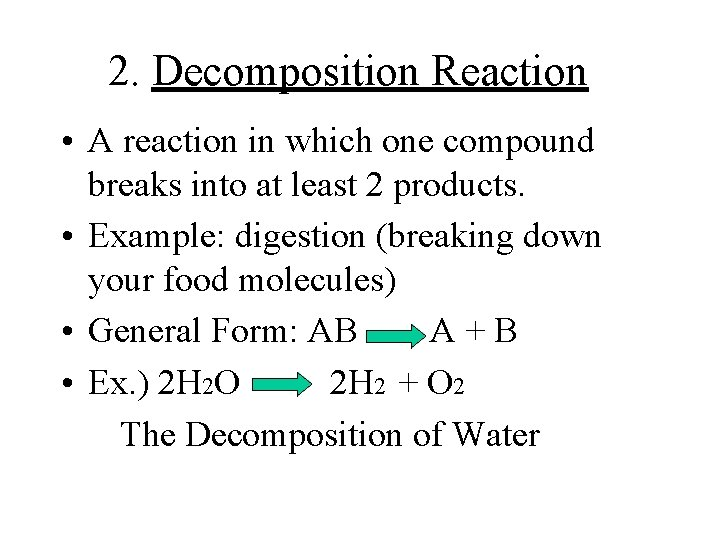2. Decomposition Reaction • A reaction in which one compound breaks into at least 2 products. • Example: digestion (breaking down your food molecules) • General Form: AB A+B • Ex. ) 2 H 2 O 2 H 2 + O 2 The Decomposition of Water3. Combustion • A reaction in which a compound and oxygen burn. • Oxygen is a reactant in every combustion reaction. • Example) the burning of methane 2 CH 4 + 4 O 2 2 CO 2 + 4 H 2 O4. Single-Displacement Reaction • A reaction in which atoms of one element take the place of atoms of another element in a compound. • General Form: XA + B AB + X • Example: Cu. Cl 2 + Al Al. Cl 3 + Cu5. Double-displacement reaction • A reaction where a compound is formed from the exchange of ions between 2 compounds. General form: AX + BY AY + BX Example: Pb(NO 3)2 + K 2 Cr. O 4 Pb. Cr. O 4 + 2 KNO 3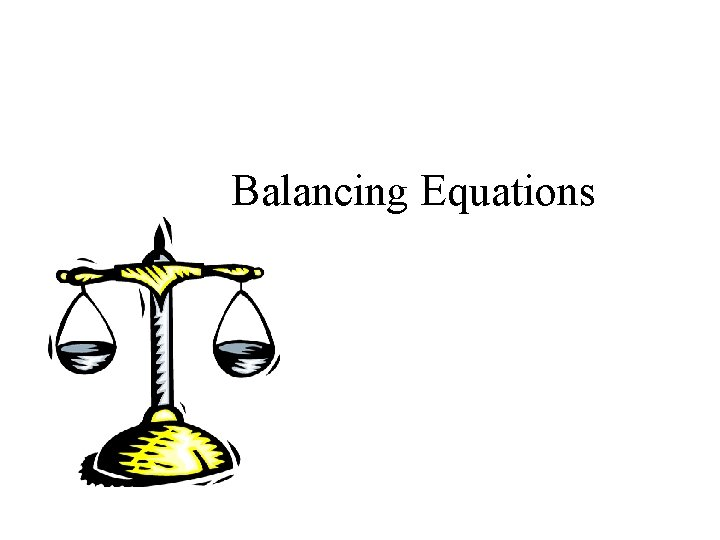Balancing Equations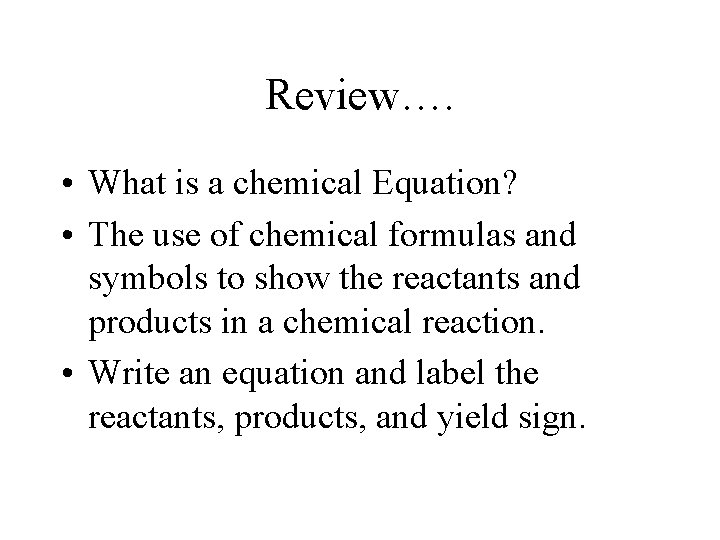Review…. • What is a chemical Equation? • The use of chemical formulas and symbols to show the reactants and products in a chemical reaction. • Write an equation and label the reactants, products, and yield sign.Why do we need to balance equations? • Equations must be balanced to account for the Conservation of Mass!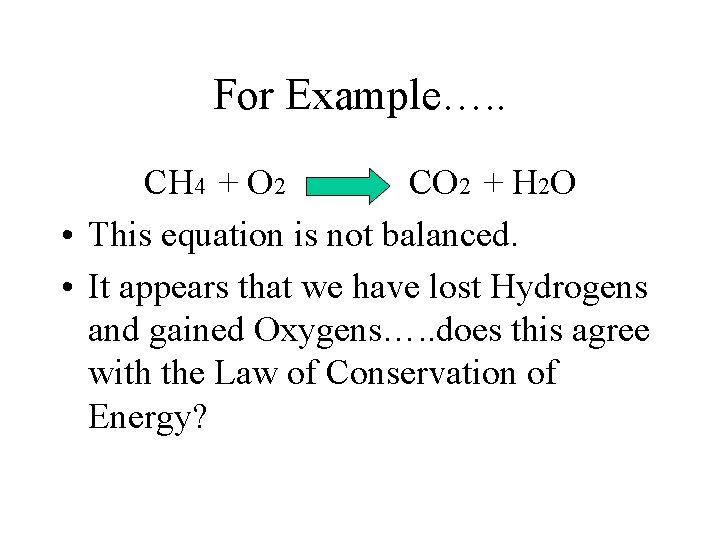For Example…. . CH 4 + O 2 CO 2 + H 2 O • This equation is not balanced. • It appears that we have lost Hydrogens and gained Oxygens…. . does this agree with the Law of Conservation of Energy?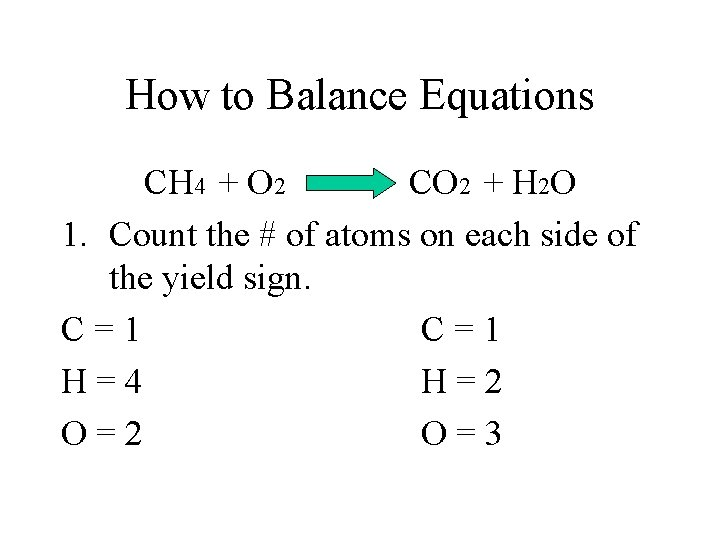How to Balance Equations CH 4 + O 2 CO 2 + H 2 O 1. Count the # of atoms on each side of the yield sign. C=1 H=4 H=2 O=3Now what? You can NOT change the subscripts! 2. Place Coefficients to balance the equation. Coefficient = a # in front of atoms or compounds.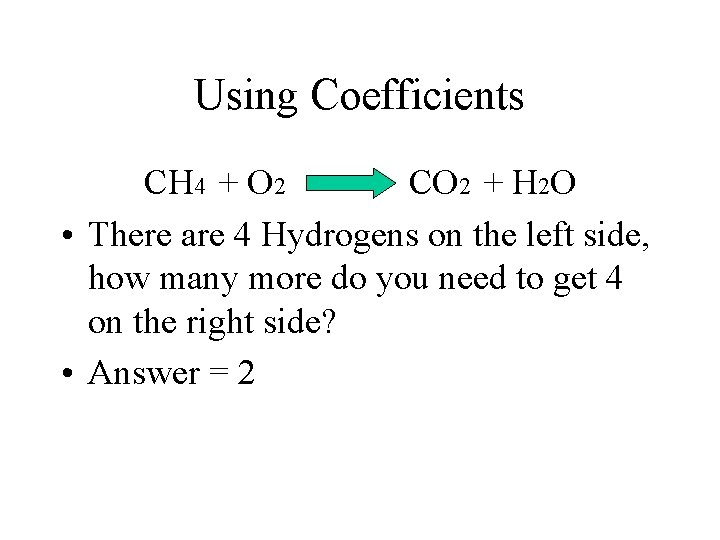Using Coefficients CH 4 + O 2 CO 2 + H 2 O • There are 4 Hydrogens on the left side, how many more do you need to get 4 on the right side? • Answer = 2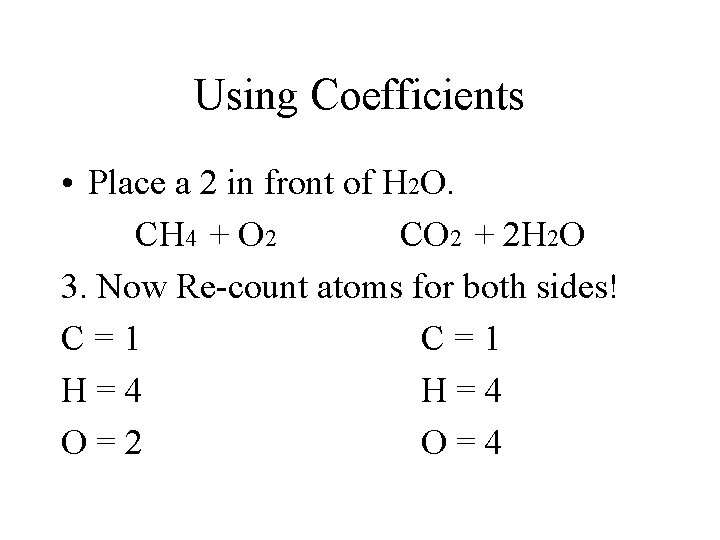Using Coefficients • Place a 2 in front of H 2 O. CH 4 + O 2 CO 2 + 2 H 2 O 3. Now Re-count atoms for both sides! C=1 H=4 O=2 O=4How to Balance the Oxygen • We need to add another Coefficient. CH 4 + O 2 CO 2 + 2 H 2 O • Oxygen needs 2 more on the left side. • Place a 2 in front of the O 2.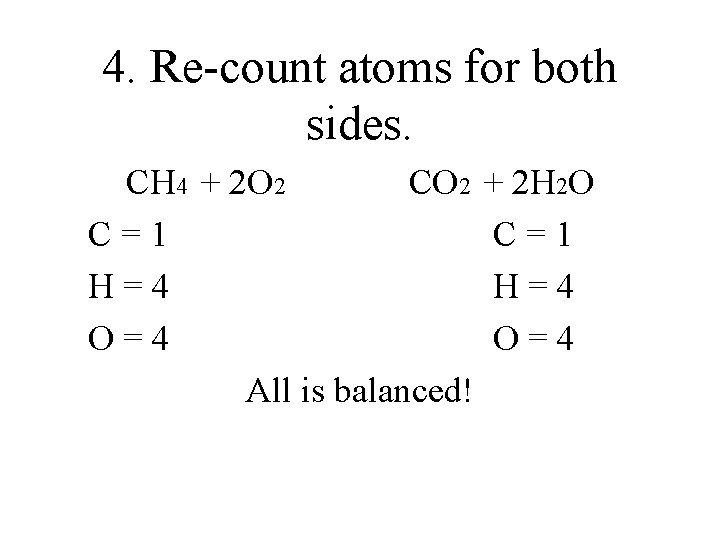4. Re-count atoms for both sides. CH 4 + 2 O 2 CO 2 + 2 H 2 O C=1 H=4 O=4 All is balanced!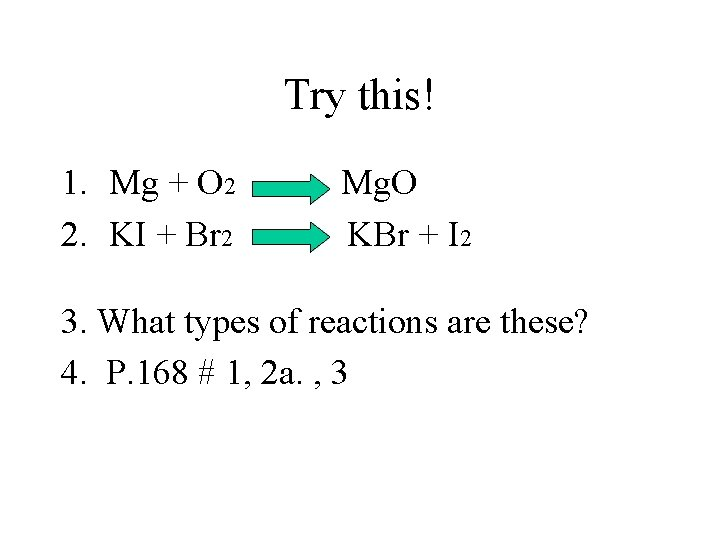Try this! 1. Mg + O 2 2. KI + Br 2 Mg. O KBr + I 2 3. What types of reactions are these? 4. P. 168 # 1, 2 a. , 3